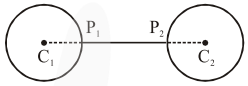# The minimum distance between

Question:

The minimum distance between any two points $P_{1}$ and $P_{2}$ while considering point $P_{1}$ on one circle and point $\mathrm{P}_{2}$ on the other circle for the given circles' equations

$x^{2}+y^{2}-10 x-10 y+41=0$

$x^{2}+y^{2}-24 x-10 y+160=0$ is

Solution:

Given $\mathrm{C}_{1}(5,5), \mathrm{r}_{1}=3$ and $\mathrm{C}_{2}(12,5), \mathrm{r}_{2}=3$

Now, $C_{1} C_{2}>r_{1}+r_{2}$

Thus, $\left(P_{1} P_{2}\right)_{\min }=7-6=1$